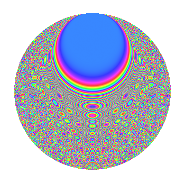# Properties

 Label 945.2.bsLevel 945 Weight 2 Character orbit bs Rep. character $$\chi_{945}(16,\cdot)$$ Character field $$\Q(\zeta_{9})$$ Dimension 576 Newforms 2 Sturm bound 288 Trace bound 1

# Learn more about

## Defining parameters

 Level: $$N$$ = $$945 = 3^{3} \cdot 5 \cdot 7$$ Weight: $$k$$ = $$2$$ Character orbit: $$[\chi]$$ = 945.bs (of order $$9$$ and degree $$6$$) Character conductor: $$\operatorname{cond}(\chi)$$ = $$189$$ Character field: $$\Q(\zeta_{9})$$ Newforms: $$2$$ Sturm bound: $$288$$ Trace bound: $$1$$ Distinguishing $$T_p$$: $$2$$

## Dimensions

The following table gives the dimensions of various subspaces of $$M_{2}(945, [\chi])$$.

Total New Old
Modular forms 888 576 312
Cusp forms 840 576 264
Eisenstein series 48 0 48

## Trace form

 $$576q$$ $$\mathstrut +\mathstrut 6q^{6}$$ $$\mathstrut +\mathstrut 12q^{9}$$ $$\mathstrut +\mathstrut O(q^{10})$$ $$576q$$ $$\mathstrut +\mathstrut 6q^{6}$$ $$\mathstrut +\mathstrut 12q^{9}$$ $$\mathstrut +\mathstrut 12q^{11}$$ $$\mathstrut +\mathstrut 54q^{14}$$ $$\mathstrut -\mathstrut 48q^{17}$$ $$\mathstrut -\mathstrut 24q^{21}$$ $$\mathstrut +\mathstrut 18q^{23}$$ $$\mathstrut +\mathstrut 120q^{24}$$ $$\mathstrut +\mathstrut 18q^{29}$$ $$\mathstrut -\mathstrut 36q^{33}$$ $$\mathstrut +\mathstrut 78q^{36}$$ $$\mathstrut -\mathstrut 120q^{38}$$ $$\mathstrut -\mathstrut 24q^{39}$$ $$\mathstrut -\mathstrut 12q^{41}$$ $$\mathstrut -\mathstrut 30q^{42}$$ $$\mathstrut +\mathstrut 18q^{45}$$ $$\mathstrut +\mathstrut 36q^{47}$$ $$\mathstrut -\mathstrut 132q^{48}$$ $$\mathstrut +\mathstrut 36q^{49}$$ $$\mathstrut -\mathstrut 36q^{51}$$ $$\mathstrut -\mathstrut 30q^{54}$$ $$\mathstrut -\mathstrut 120q^{56}$$ $$\mathstrut -\mathstrut 24q^{57}$$ $$\mathstrut -\mathstrut 60q^{59}$$ $$\mathstrut +\mathstrut 18q^{61}$$ $$\mathstrut -\mathstrut 162q^{62}$$ $$\mathstrut -\mathstrut 96q^{63}$$ $$\mathstrut -\mathstrut 288q^{64}$$ $$\mathstrut -\mathstrut 6q^{65}$$ $$\mathstrut +\mathstrut 30q^{68}$$ $$\mathstrut -\mathstrut 18q^{70}$$ $$\mathstrut +\mathstrut 24q^{71}$$ $$\mathstrut -\mathstrut 204q^{72}$$ $$\mathstrut +\mathstrut 72q^{73}$$ $$\mathstrut -\mathstrut 156q^{74}$$ $$\mathstrut -\mathstrut 90q^{77}$$ $$\mathstrut +\mathstrut 96q^{78}$$ $$\mathstrut -\mathstrut 18q^{79}$$ $$\mathstrut -\mathstrut 84q^{80}$$ $$\mathstrut +\mathstrut 12q^{81}$$ $$\mathstrut +\mathstrut 60q^{83}$$ $$\mathstrut -\mathstrut 108q^{84}$$ $$\mathstrut +\mathstrut 36q^{85}$$ $$\mathstrut +\mathstrut 6q^{86}$$ $$\mathstrut -\mathstrut 60q^{87}$$ $$\mathstrut +\mathstrut 36q^{91}$$ $$\mathstrut -\mathstrut 48q^{92}$$ $$\mathstrut -\mathstrut 24q^{93}$$ $$\mathstrut -\mathstrut 36q^{94}$$ $$\mathstrut +\mathstrut 216q^{96}$$ $$\mathstrut -\mathstrut 48q^{98}$$ $$\mathstrut -\mathstrut 108q^{99}$$ $$\mathstrut +\mathstrut O(q^{100})$$

## Decomposition of $$S_{2}^{\mathrm{new}}(945, [\chi])$$ into irreducible Hecke orbits

Label Dim. $$A$$ Field CM Traces $q$-expansion
$$a_2$$ $$a_3$$ $$a_5$$ $$a_7$$
945.2.bs.a $$276$$ $$7.546$$ None $$0$$ $$-6$$ $$0$$ $$0$$
945.2.bs.b $$300$$ $$7.546$$ None $$0$$ $$6$$ $$0$$ $$0$$

## Decomposition of $$S_{2}^{\mathrm{old}}(945, [\chi])$$ into lower level spaces

$$S_{2}^{\mathrm{old}}(945, [\chi]) \cong$$ $$S_{2}^{\mathrm{new}}(189, [\chi])$$$$^{\oplus 2}$$